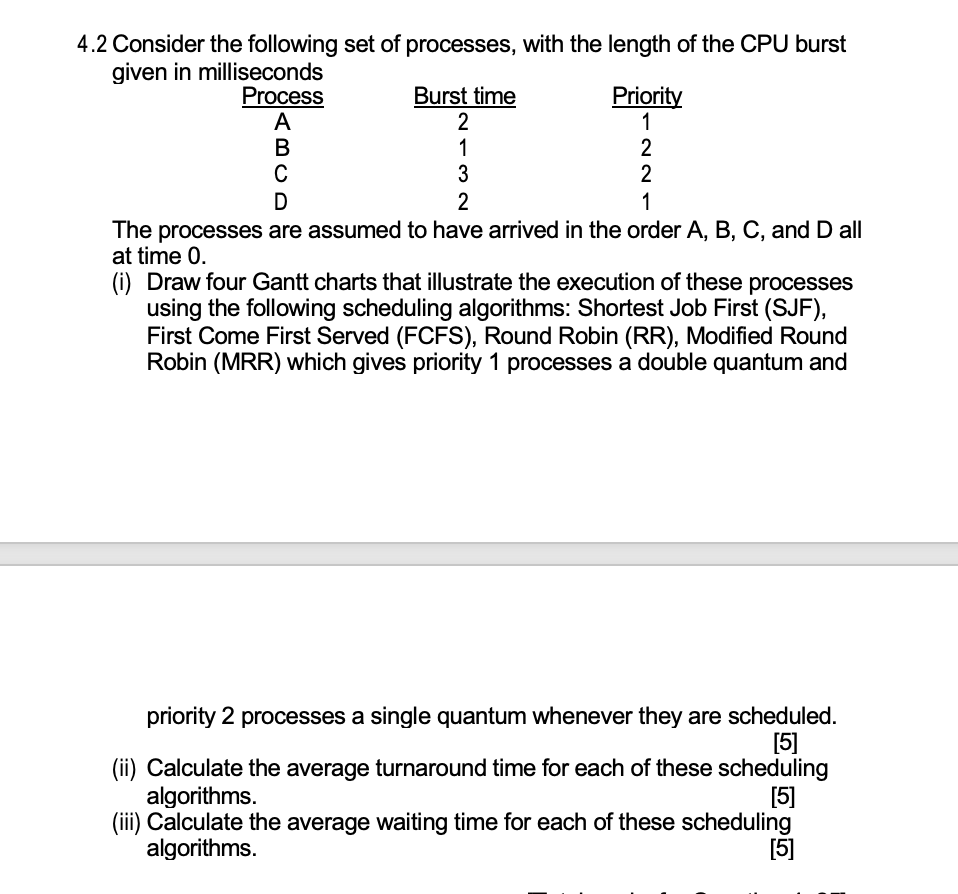Home / Expert Answers / Computer Science / 2-consider-the-following-set-of-processes-with-the-length-of-the-cpu-burst-g-pa667

# (Solved): 2 Consider the following set of processes, with the length of the CPU burst g ...???????

2 Consider the following set of processes, with the length of the CPU burst given in milliseconds The processes are assumed to have arrived in the order , and all at time 0 . (i) Draw four Gantt charts that illustrate the execution of these processes using the following scheduling algorithms: Shortest Job First (SJF), First Come First Served (FCFS), Round Robin (RR), Modified Round Robin (MRR) which gives priority 1 processes a double quantum and priority 2 processes a single quantum whenever they are scheduled.  (ii) Calculate the average turnaround time for each of these scheduling algorithms.  (iii) Calculate the average waiting time for each of these scheduling algorithms. 

We have an Answer from Expert